InfoQ Homepage Articles Understanding Monads. A Guide for the Perplexed

# Understanding Monads. A Guide for the Perplexed

### Key Takeaways

• It's worth avoiding explicitly handling stateful values.
• Using monads, you can remove the explicit handling of stateful values from your code.
• In order to qualify as a monad type, a type must have a particular kind of function (named “bind”) associated with it.
• With a monad’s bind function, a stateful value gets passed from one monad to another, always staying inside of monad (instead of being handled explicitly).
• Many different kinds of problems can be solved using monads.

With the current explosion of functional programming, the "monad" functional structure is once again striking fear into the hearts of newcomers. Borrowed from the field of category theory in mathematics, and introduced into programming languages in the 1990s, monads are a fundamental construct in pure functional languages like Haskell.

Here's what most newcomers know about monads:

• A monad is useful for doing input and output.
• A monad is useful for other things besides input and output.
• A monad is difficult to understand because most of the articles about monads go into too much detail or too little detail.

The third bullet motivates me to write this article -- the hundredth (or maybe even the thousandth) article that introduces readers to monads. With any luck, you'll finish this article feeling that monads aren't so scary.

## The State of a System

In a computer program, the word "state" describes the global variables, the input, the output, and anything else that's not local to a particular function. Here are some things to remember about a program's state:

• Its values persist from the execution of one function to another.
• It's available to more than one function.
• It can be mutable. If so, its value is time-dependent.

State is tricky to manage because no single function owns the state. Consider this scenario:

• Function Number 1 obtains a value from the state and uses that value in executing its instructions. In the meantime, Function Number 2 modifies that value. As a result, Function Number 1 makes decisions based on a value that's not up-to-date.
• To make matters worse, Function Number 1 uses its stale data to perform a calculation, and then replaces the state value with a new, inaccurate value. Now Function Number 1's error has become contagious, spreading from within the function to the entire global system.

One way or another, the state of the system is a function of time, so time is an extra dimension that we have to worry about. We can't really ask "What's the value of x?" Instead, we have to ask "What's the value of x at time t?". This adds a dimension of complexity that makes it difficult to reason about the code. So the bottom line is ...

No state: good!

## Expressions and Actions

An expression is a piece of text that has a value. Consider, for example, the following code:

     x = 5
     y = x + 7
     x = y + 1

The first occurrence of x is an expression with the value 5. The last occurrence of x is an expression with the value 13. The code contains other expressions. For example, in the middle line, x + 7 is an expression whose value is 12.

In most computer languages, a command to read from the keyboard is an expression, and that expression has a value. Consider the following statement:

     x = nextInput()

You'll find statements of this kind in Java, C++, and in many other programming languages. Now imagine that the user types the number 5. Then nextInput() is an expression whose value is 5. Execution of the statement assigns the value of the nextInput() expression (the value 5) to the variable x. If x is part of the program's state, then this statement modifies that state. And as we've already seen, modifying a system's state can be dangerous.

In our nextInput() example, the value of nextInput()is time-dependent. The first time you execute nextInput(), the value of the nextInput() expression might be 5. Later, when you execute nextInput() a second time, the same expression's value might be 17. In a loosely typed language, the value of nextInput() might change from 5 to "Hello, world".

To eliminate time-dependency, we stop using nextInput() and replace it with a function that I'll call doInput. As an expression, the value of doInput() isn't 5 or 17 or "Hello, world". Instead, the value of doInput() is an action. It's an action that obtains input from the keyboard at runtime.

An action is something that may or may not happen. Reading from the keyboard is an action. Writing "Hello, world" to the screen is an action. Opening the file found at "/Users/barry/myfile.txt" is an action. Forming a connection to http://www.infoq.com is an action. An action is a computation of some kind. Typically, an action’s details aren’t spelled out in detail in a program’s source code. Instead, an action is a run-time phenomenon.

In many languages, when you think about types, you think about integer, float, string, boolean, and other such things. You probably don’t think of action as a type. But when we do monadic I/O, the value of an expression such as doInput() is an action. To say it in a slightly different way, the value returned from a call to doInput() is of an action type.

With this way of thinking, the value of doInput() isn't time dependent. No matter where the expression doInput() appears in a program, that expression always has the same value. Its value is the action that obtains input from the keyboard.

In terms of state versus lack-of-state, we've made progress.

## You Can't Do Much with an Action Value

We have a slight problem. We want to do something useful with an expression whose value is an action. How can we do that? If an action gets the number 5 from the keyboard, we can't add 1 to the action.

     x = doInput()
     print(x + 1)

In this language-agnostic code, the variable x refers to an action. An action is of one type, and the value 1 is of a completely different type. So the expression x + 1 makes no more sense than adding 1 to an action that restarts the computer. The expression restart + 1 is pure  nonsense!

What if you don't want to add 1 to the user's input? Does the following code now make sense?

     x = doInput()
     print(x)

No, it doesn't. The statement x = doInput() assigns an action to x, so the statement print(x) is trying to display an action. And what does an action look like when you try to display it on a computer screen?

You might argue that, in a weakly typed language, you can blur the differences between input actions and the values that they obtain from the keyboard. Just as 2 == "2" is true in JavaScript, 5 == the_action_obtaining_5 might be true in some weakly typed functional language. But under the hood, some kind of processing is required to get 5 from the_action_obtaining_5. And besides, if you lose the distinction between 5 and the_action_obtaining_5, then there's very little difference between doInput() and the original nextInput();  you're back to where you started with the input function call being a time-dependent expression. That's not where you want to be.

## Creating a Chain

So the case is clear; we can't print the value of the doInput() expression. But what if we could  cleverly chain actions and follow the doInput() action with another action whose effect is to print? That, dear reader, would be a monad.

A doInput() action has something to do with a value such as 5, a value that the user enters on the keyboard. When we deal with doInput(), we're most interested in the number 5 that the user types, not in the doInput() action itself.

Let's take a closer look. If a program keeps track of the inventory in a warehouse, the input number 5 might represent the number of boxes on a shelf. The value 5 is pertinent in the problem domain. If you walk up to someone who manages the warehouse and say "You have 5 boxes," that person knows what you mean. On the other hand, the value of the doInput()expression is an action that has no meaning for people who manage inventories. The doInput()action is an artifact of the way we process inventories. So in this article, I distinguish the pertinent value(s) associated with an action from the action itself. To emphasize the action's lack of pertinence, I refer to the action itself as the artifact.

Here's a recap. When you execute doInput() and the user enters the number 5,

• The action that receives input is what we will call the artifact.
• The number 5 is what we will call the pertinent value.

This pertinent/artifact terminology won’t work nicely with each and every monad, but it helps me explain what monads are all about. To help even more, I make the pertinent value an integer value in most of this article's examples.

If I'm being sloppy, I think of the doInput() action as a container that has a pertinent value such as 5 bubbling around inside of it. The container metaphor is useful because, once a value is associated with a monad, we like to keep that value attached to the monad. We don't like to let the value out of its monad container. Please remember that these pertinence and container analogies break down when you become serious about working with monads. Even so, the analogies are extremely useful for developing intuitions about monads.

So here's our challenge: we must formalize a way of using the pertinent user input that's associated with the doInput() action. (In this article, the word "formalize" doesn't mean "to make absolutely rigorous." Instead, it means "to make somewhat precise using a slightly simplified description in plain English.")

## Some Types are Monads; Others Aren't

In a typical programming language, you might have an integer type, a float type, a boolean type, a string type, and many different kinds of compound types. You can describe a type as either being, or not being, a monad type. What kind of a type is a monad type?

For starters, a monad type has a pertinent value or many pertinent values associated with it. Here are some examples of types with pertinent values:

1. The I/O Action type:
For an input-from-keyboard action, the pertinent value is whatever the user enters. The artifact is the action itself.

2. The List type:
Imagine a list of values: [3, 17, 24, 0, 1]. In this list, the pertinent values are 3, 17, 24, 0, and 1. The artifact is the fact that the values are gathered to form a list. If you don't like thinking about a list, think about an array, a vector, or about any other collection structure from your favorite programming language.

3. The Maybe type:
The null value is problematic in many languages, and functional programming has a workaround. In my simplified scenario, a Maybe value contains either a number (such as 5) or a Nothing indicator. The Maybe would contain Nothing instead of a value if a calculation failed to determine a value.

Languages such as Java and Swift have Optional types. An Optional is similar to our Maybe type.

For a calculation that yields a Maybe value, the pertinent value is either a number or the special Nothing indicator. The artifact is the notion that the calculation's result isn't simply a number.

4. The Writer type:
A Writer is a function that, as part of its work, produces some information that’s written to an ongoing log. Imagine, for example, a fancy squaring function with a secondary value attached to it:

square(6) = (36, "false")

The number 36 is 6*6. The word "false" indicates that the answer, 36, is not divisible by 10. After several applications of different Writer functions, the log might look like this:

"false true false"

In this example, the pertinent value is a numeric result such as 36. The artifact is the string value ("true" or "false") and bundling of the numeric result with the string value.

For each type, formalizing the use of the type’s pertinent values presents a bit of a challenge. In particular:

1. For the I/O Action type:
You have an action, and some user input is associated with that action. You can't just add 1 to that action and you can't echo that action on the computer screen. The action doesn't have the correct type. You have to define how you're going to do something with the action's pertinent value.

2. For the List type:
You have a list with the numbers; in our example 3, 17, 24, 0, and 1, inside of it. You can't do a numeric calculation on the list itself. The list doesn't have the correct type. You have to define how you're going to do something (such as "+ 1") with each of the numbers inside the list.

3. For the Maybe type:
Imagine two Maybe values named maybe1 and maybe2. The maybe1 value has Nothing associated with it, and the maybe2 value has the number 5 associated with it. The maybe1 value came from some unsuccessful calculation, and the maybe2 value came from some calculation in which 5 was the result.

Can you add 1 to the maybe1 value? No. The Nothing value is associated with maybe1, so 1 + maybe1 is meaningless.

Can you add 1 to the maybe2 value? For the purpose of learning about monads, my answer is still no. The 5 value is associated with maybe2, but maybe2 isn't the same as 5. The maybe2 value isn't a number -- it's an artifact structure that happens to have 5 associated with it. So 1 + maybe2 is meaningless.

The missing link is that you have to define how you're going to do something with the pertinent value that's associated with the Maybe value.

4. For the Writer type:
Start with some ordinary functions that help you find ((6 * 6) + 4) / 5.

square(6)      = 36 plus4(36)      = 40 dividedBy5(40) = 8

You can chain these functions together to get the result that you want:

dividedBy5(plus4(square(6))) = 8

But if each function is a Writer, and each function's result includes a divisible-by-10 indicator, the story is different:

The accumulated log contains:

     square(6)      = (36, "false")      "false"

     plus4(36)      = (40, "true")       "false true"

     dividedBy5(40) = ( 8, "false")      "false true false"

You can't apply the plus4 function to the pair that the square function returns.

     plus4(square(6)) is

plus4((36, "false") which is meaningless

Instead, you apply the plus4 function to the pertinent value from the square function's execution. Similarly, you apply the dividedBy5 function to the pertinent value from the plus4 function's execution.

With one simple rule, you can formalize once and for all, a way to apply each function call to the previous call's pertinent value. Which brings us to the bind function.

## For a Type to be a Monad, It Must Have a Bind Function

In most programming languages, types have their own functions. For example, an integer type has its +, -, *, and / functions. A string type has its concatenate function. A boolean type has its or, and, and not functions.

To qualify as a monad, a type must have a function that uses the monad's pertinent value or values, and the function must have a particular form. Let’s see what that form is.

The first candidate for the function's form (an incorrect idea) is for the function (which I'll call badIdea) to isolate the pertinent value from the monad. For example, the badIdea function might grab the user's input from an action. If you call doInput() and the user types the number 5, then badIdea(doInput())is 5. Having applied the badIdea function, you can print the badIdea() call's result value. You can even add 1 to the badIdea() call's result value.

     x = badIdea(doInput())
     print(x)
     y = x + 1

Now you're back where you started before we raised the nextInput() function's time dependency issue. The expression badIdea(doInput()) has all the undesirable features of the original nextInput() function. Execute badIdea(doInput()) once, and as an expression, its value might be 5. Execute badIdea(doInput()) another time and its value might become 17. In a loosely typed language, the value of badIdea(doInput()) might change from 5 to "Hello, world".

With the badIdea function, you grab the pertinent value from the doInput() action and do anything you want with that value. Instead of implementing that bad idea, let’s grab the pertinent value from the doInput() action and create another action that does something useful with that value. This is important: you follow the doInput() action with another action. When you formalize this idea, the action type becomes a monad type. So let's start formalizing the idea:

We begin with two things: an action and a function. For example,

• The action, doInput(), gets a number from the keyboard.
• The function, doPrint, takes a number, and yields an action that writes that number on the screen.

doPrint(5) = an action that writes 5 on the screen
doPrint(19) = an action that writes 19 on the screen

Figure 1 illustrates this scenario. In this figure, each gear drawing stands for an action of some kind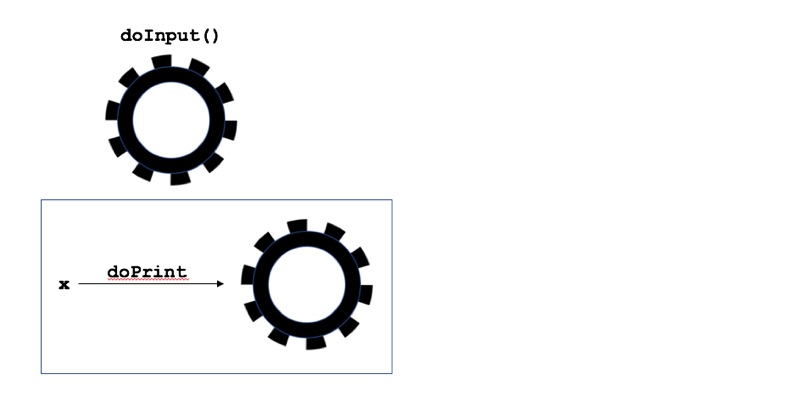I can describe doPrint as a from-number-to-action function. When you do functional programming, functions of this kind crop up naturally.

Let’s pause here for a word on my notation; consider two expressions such as opSystem without parentheses, and opSystem() with parentheses. The opSystem expression’s value is a particular function -- the function that reaches out and discovers what operating system is currently running. But the opSystem() expression’s value is a name -- something like "Linux" or "Windows 10". In short,

• opSystem stands for a function, and
• opSystem() stands for the value that’s returned from a call to the opSystem function..

With that in mind, notice the difference between “the action doInput()” with parentheses and “the function doPrint” without parentheses.  Both the doInput and doPrint functions return values, and in both cases, the values are actions. When I write “the action doInput(),” I’m referring to the value returned by the doInput() function call.  When I write “the function doPrint,” I’m referring to the doPrint function itself, not to the function’s return value. This accounts for the difference in the way I illustrate doInput() and doPrint in Figure 1. To illustrate doInput(), I  draw a gear, which is supposed to remind you of an action. To illustrate doPrint, I draw an arrow, which reminds you of a function. When you’re thinking about monads, it helps to keep such issues front and center in your thinking.

With the doInput() action and the doPrint function, we need one more piece to make a monad; we need a way of gluing doInput() and doPrint together. More precisely, we need a formula for taking any action, A, and any from-number-to-action function, f, and combining them to create a new action. We give this formula the name bind. See Figure﻿ 2.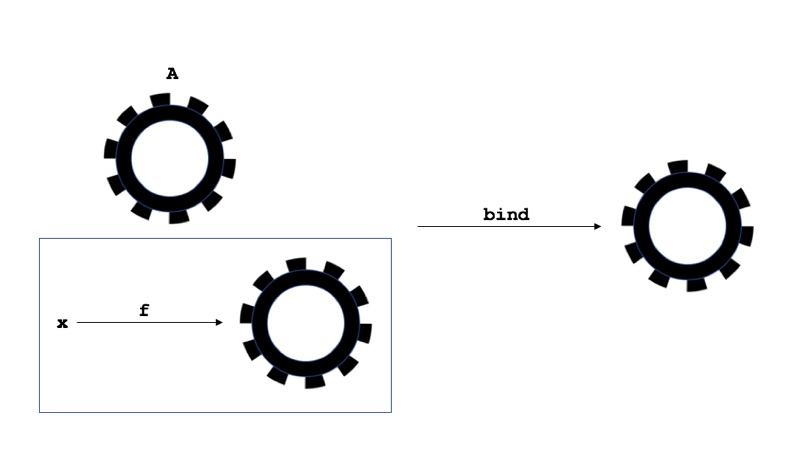In the previous paragraph, I call bind a formula, but bind is really another function.

bind(A,f) = an action of some kind

The bind function is a higher-order function because the bind function takes a function as one of its arguments. If you're not used to thinking about functions of functions, the bind function can make your head spin.

For input and output, the bind function must be a general rule that takes, as its parameters, any I/O action A and any from-number-to-action function f. The bind function must return a new I/O action. For example:

• doInput() = an action that gets a number from the keyboard
doPrint(x) = an action that writes the value of x on the screen

bind(doInput(),doPrint) =
an action that writes doInput()'s pertinent value on the screen

See Figure 3.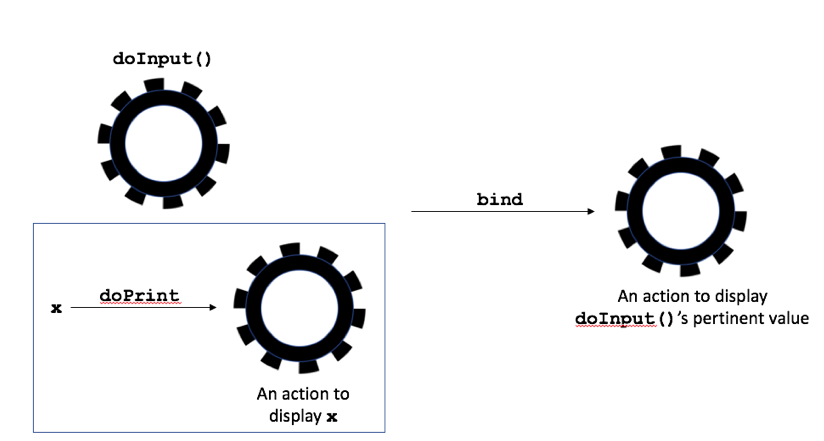Let's change the example a tiny bit:

• Let doPrintPlus1(x) = an action that writes the value of x+1 on the screen

bind(doInput(),doPrintPlus1) =
an action that writes (doInput()'s pertinent value)+1 on the screen

See Figure 4.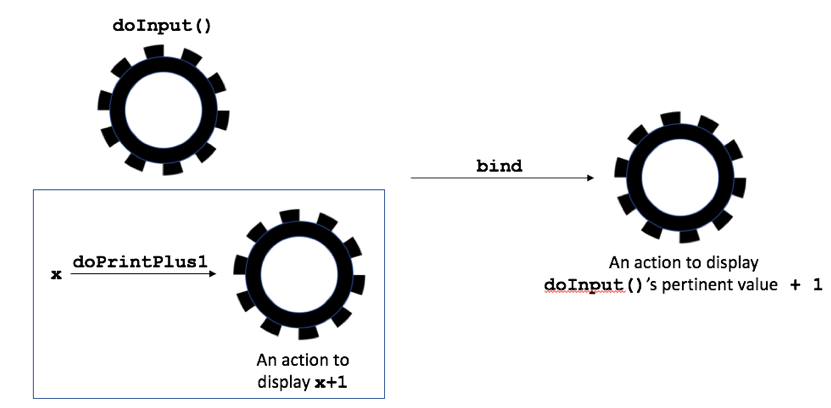In general, for an input/output action, A, and a from-number-to-action function, f:

bind(A,f) =  an action that applies f to A's pertinent value

See Figure 5.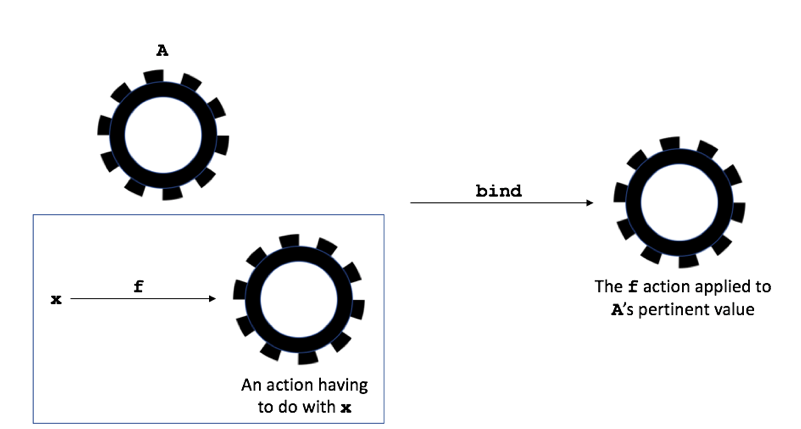If you find the description of bind to be confusing, you're not alone. Higher-order functions aren't easy to understand.

In the Haskell programming language, the bind function plays such an important role that a bind operator, >>=, is built into the language. In fact, many features for dealing with monads are baked directly into Haskell.

## No More Time Dependency

Let's revisit the doInput(),doPrint scenario that's illustrated in Figure 3.

bind(doInput(),doPrint) =
an action that writes doInput()'s pertinent value on the screen

No part of the bind(doInput(),doPrint) expression has a time-dependent value. No matter when this expression appears in your code,

• doInput() is always the same action -- the action that gets a number from the keyboard.
• doPrint is always the same from-number-to-action function.
• The entire expression, bind(doInput(),doPrint), is always the same action -- the action that writes a number to the screen.

The value that the user types on the keyboard changes from one moment to the next, but no part of the bind(doInput(),doPrint) expression represents that value. We haven't eliminated all side effects, but we've eliminated any explicit mention of the system's state in our code. Once the user types a number on the keyboard, that number gets passed like a hot potato from one action to another. None of the code's variables stand for the value that the user typed.

## It’s Not Always about Numbers and Input/Output Actions

In the examples that we’ve seen so far,

• The pertinent value of doInput() is a number, and the argument type of doPrint is also a number.
• The type of doInput() is an action, and the return type of doPrint is an action.

The bind function tells you how to combine doInput() and doPrint in order to get a brand new action.

Some monads don’t have anything to do with numbers or with input/output actions. So, let’s state our observations about the doInput, doPrint and bind functions in a slightly more general way:

• The argument type of doPrint is the same as the pertinent value type of doInput().
• The return type of doPrint is the same as the type of doInput().

The bind function tells you how to combine doInput() and doPrint in order to get a brand new value. That new value has the same type as the original doInput() value’s type.

## More Monad Types

Any type that has a bind function (and one other function that I describe toward the end of this article) is a monad. With the bind function for input/output that I describe in the previous few paragraphs, the input/output action type becomes a monad. The list, Maybe, and Writer types that we covered earlier are also monad types. Here's how it works with the list type:

Start with two things: a list, L, and a function, f.  Again, the function f is of a certain kind.

• As its argument, f takes a value whose type is the same as an element in the list.
• For its result, f yields a list.

If the list contains numbers, f is a from-number-to-list function. Here's a formula (a definition of bind) that takes any list and any from-number-to-list function, and combines them to create a new list:

    bind(L,f) =  the new list that you get by applying f to each of
L's elements and then flattening the resulting list of lists

Let's look at an example:

• Let f(x) = the list [squareRoot(x),-squareRoot(x)]

As is required, f is a from-number-to-list function.

Let L = [4,25,81]

From our definition of bind for lists:

bind(L,f) = the flattening of [[2,-2], [5,-5], [9,-9]]

          = [2,-2,5,-5,9,-9]

The bind function gives you all the possible results of applying the function f to any of the elements in list L. See Figure 6.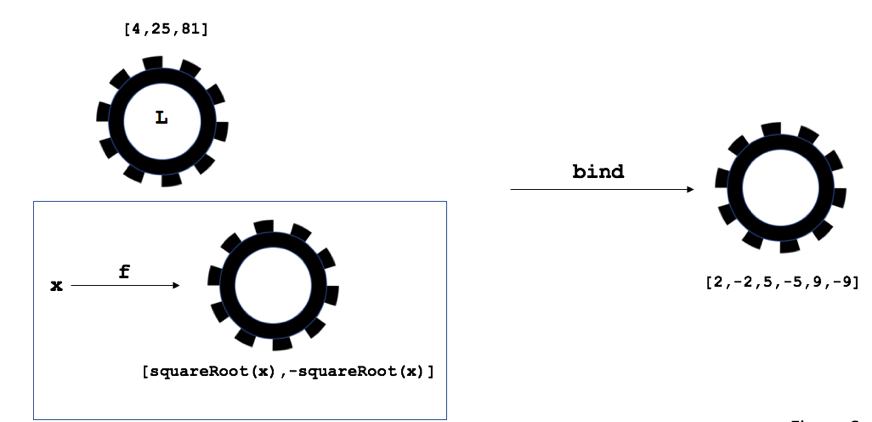• Here's an example that doesn't involve numbers. Let x be a book, and let f(x) = the list of authors of that book

For example,

f(C_Programming_Lang) = [Kernighan,Ritchie]

In this example, f is a from-book-to-list function. From our definition of bind for lists:

bind([C_Programming_Lang, Design_Patterns], f) =

the flattening of
[[Kernighan,Ritchie],[Gamma,Helm,Johnson,Vlissides]] =
[Kernighan,Ritchie,Gamma,Helm,Johnson,Vlissides]

We've managed to get a list of authors from a list of books. See Figure 7.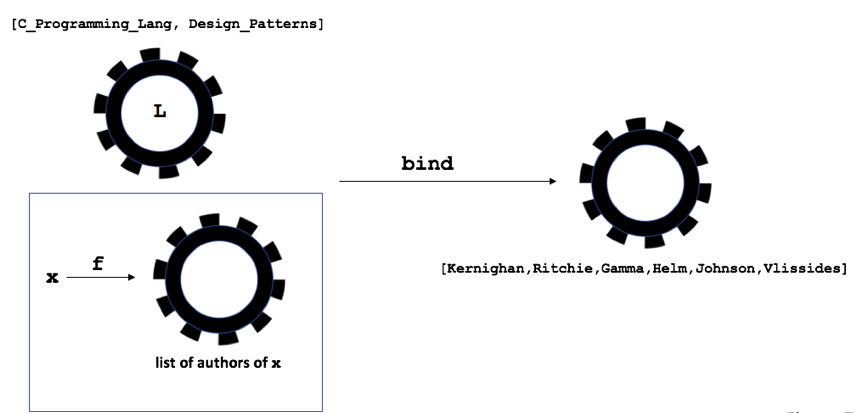Notice the similarities among Figures 2 through 7:

• On the left side of the diagram, you have a monad value (e.g., an action, a list) and a function. The function takes a pertinent value and yields a monad value.
• In the middle left, you have an arrow indicating the application of a bind function.
• On the right side of the diagram you have a brand new monad value.

How about a bind function for the Maybe type? Let m be a Maybe value (containing a number or Nothing), and let f be a function that takes a number and yields a Maybe value. The value of bind(m,f)depends what's inside the Maybe value:

         bind(m,f) =   either
f(m's pertinent value)    if m doesn't contain Nothing
or
Nothing                                            if m contains Nothing

Let's line up some examples.

Let maybe1 contain Nothing
Let maybe2 contain 5
Let maybe3 contain 0
Let f(x) = a Maybe value containing 100 / x

Then

bind(maybe1,f) = Maybe value that contains Nothing
     bind(maybe2,f) = Maybe value that contains 20
     bind(maybe3,f) = Maybe value that contains Nothing

Once again, the bind function's arguments are a monad (in this case, a Maybe value) and a function. The function's argument is a monad's pertinent value, and the function returns another monad.

Of course, we can create a bind function for the Writer monad.

bind((aNumber,aString), f) =

(the numeric part of f(aNumber), aString+the string part of f(aNumber))

Some examples help bring the point home. Recall our square, plus4, and dividedBy5 functions -- the functions whose return values include a divisible-by-10 indicator.

bind((36, "false"), plus4) = (40, "false true")  See Figure 8.

bind((40, "false true"), dividedBy5) = (8, "false true false")

When you apply bind, the string part of the result includes the strings from the earlier calculations. The string part is running log of all the calculations.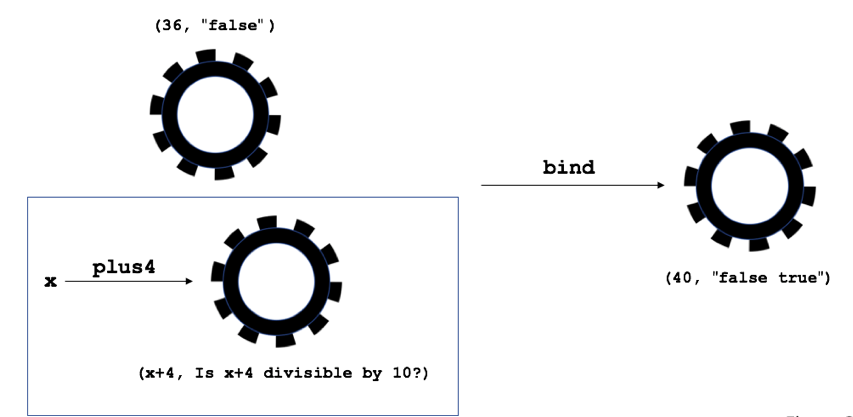## A Monad Needs One Additional Function

The bind function may not be easy to understand, but the other function that a monad must have is fairly simple. I call it the toMonad function.

• The toMonad function takes as its argument a pertinent value.
• The toMonad function returns a monad.

Roughly speaking, toMonad returns the simplest monad that can possibly contain the given pertinent value. (There's a more precise definition involving toMonad's interaction with bind, but I won't go into that. If you’re curious, visit https://en.wikibooks.org/wiki/Haskell/Understanding_monads.)

1. For the I/O Action monad, toMonad(aValue) = an action whose pertinent value is aValue but whose effect is to do nothing at all.

2. For the list monad, toMonad(aValue) = the list containing the aValue as its only entry. See Figure 9.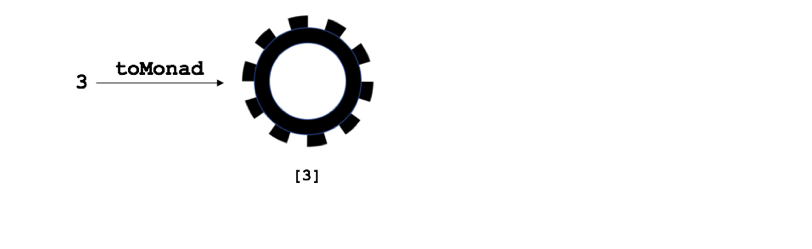3. For a Maybe monad, toMonad(number) = a Maybe value containing that number

Remember that the Maybe value containing 5 isn't exactly the same as the plain, old number 5.

4. For a Writer monad, toMonad returns a monad containing the empty string.

toMonad(number) = (number, "")

## That's It!

A monad is a type with a bind function and a toMonad function. The bind function goes mechanically from monad to monad without explicitly unveiling a monad's pertinent value.

When you think functionally, monads pop up all over the place. With the I/O action monad, none of the expressions in your code stand for the user's input. So the state of the system isn't explicitly expressed anywhere in your program. The expressions in your code aren't time-dependent. You never ask "What does x mean at this point in the program?" Instead, you ask "What does x mean once and for all in this program?"

Remember the bottom line...

No state: good!

The world that we live in is stateful, and there's nothing we can do about that. Monads don't eliminate state from a system. But monads eliminate the mention of state from your program code. That can be mighty helpful.

## About the AuthorDr. Barry Burd has authored many articles and books, including the popular "Java For Dummies", and "Android Application Development All-in-One For Dummies", both from Wiley Publishing. He’s also the instructor for O’Reilly’s Introduction to Functional Programming course. Dr. Burd has been a professor in the Department of Mathematics and Computer Science at Drew University in Madison, New Jersey since 1980. He has lectured at conferences in the United States, Europe, Australia, and Asia.

Style

## Hello stranger!

You need to Register an InfoQ account or or login to post comments. But there's so much more behind being registered.

Get the most out of the InfoQ experience.

Allowed html: a,b,br,blockquote,i,li,pre,u,ul,p

• ##### Small error

by Michael Donkhin /

• ##### Re: Small error

by Barry Burd /

• ##### Perplexing statements/errors

by Jon Kleiser /

• ##### Re: Perplexing statements/errors

by Aldrich Wright /

• ##### Re: Perplexing statements/errors

by Barry Burd /

• ##### My perplextion shifted

by Patrik Iselind /

• ##### Re: My perplextion shifted

by Barry Burd /

• ##### Small error

Your message is awaiting moderation. Thank you for participating in the discussion.

Scala is not a pure functional language, it's actually a hybrid one with many of its functional constructs expressed through objects.

• ##### Perplexing statements/errors

by Jon Kleiser /

Your message is awaiting moderation. Thank you for participating in the discussion.

1. "Execute nextInput() another time and, as an expression, its value is 17." Why 17?
2. Under Figure 7 are references to "the middle of the diagram" and "the right side of the diagram", but it's not clear to me which is which. I usually know the difference between "middle" and "right".
3. "Let maybe2 contain 0" cannot lead to "bind(maybe2,f) = a Maybe value that contains 20". Probably some typo.
This is not good enough, Dr. Burd.

• ##### Re: Perplexing statements/errors

Your message is awaiting moderation. Thank you for participating in the discussion.

1. He implied one action, that the user entered 17 the second time. I had to reread it a second time to see what you were talking about.

2. There are three gears in the diagram. The topmost left has an 'L' in it. The middle one (to the right and below it) has an arrow and a gear, the right part of the diagram is the gear to the right of the middle one. How was this confusing?

3. You're right about the last, of course.

• ##### Re: Small error

by Barry Burd /

Your message is awaiting moderation. Thank you for participating in the discussion.

You're correct, Michael. Scala isn't purely functional. That's a typo.

• ##### Re: Perplexing statements/errors

by Barry Burd /

Your message is awaiting moderation. Thank you for participating in the discussion.

Jon,
You make some good points. Thanks for catching these...

1. Let's rephrase this sentence as follows to make it clearer: "If you execute nextInput() another time, then its value as an expression might be something completely different, such as 17."

2. Let's rephrase these sentences to make them clearer:
* On the top left in the diagram, you have a monad value (e.g., an action, a list) and a function. The function takes a pertinent value and yields a monad value.
* On the bottom left, you have an arrow indicating the application of a bind function."
* On the right side if the diagram, you have a brand new monad value.
Is that better?

3. The value 0 is a typo. Here's the correction:
Let maybe2 contain 5.
Let f(x) = a Maybe value containing 100 / x.
bind(maybe2, f) = a Maybe value that contains 20

• ##### My perplextion shifted

Your message is awaiting moderation. Thank you for participating in the discussion.

I have for quite some time tried to figure out this Monad thingy and your way of describing it feels quite new to me compared to other descriptions I've read.

It seems to me that, based on the way you describe it, Monads are simply another way to compose functions of similar/same signature compared to some 'traditional' way. Something like, How you stitch together functions to form 'pipelines' of sorts.

So instead of chaining functions something like d(c(b(a()))), you do something alone the lines of compose(a,b,c,d) or map(f, [a,b,c,d) to acheive the same thing. I cannot see that any one of these have any advantage over the others here, yet. It does get tricky though when the functions' in-parameters doesn't match their out-parameters. Is that also something that is 'solved' by Monads?

The main driver for Monads as i understand it is to get out of the 'must' of saving a global state. Am i on the right track?

I do have a hard time picturing this 'action' type that you speak of in your article. Are there any concrete examples of these 'actions' that are easier to relate to that you can provide? How is for example list an action?

My reason for hunting Monads is to see if there is something useful in Monads for me when designing my programs. Today i'm exclusively using imperative programming languages, but as i understand it Monads are not something that is exclusive to functional programming languages.

• ##### Re: My perplextion shifted

by Barry Burd /

Your message is awaiting moderation. Thank you for participating in the discussion.

Patrik,
I can try to help you out of your newly found perplexed state.

You write "Monads are simply another way to compose functions of similar/same signature compared to some 'traditional' way." That's sort-of true. When you have a monad, the monad comes with its own definition of the bind function. The bind function gets us past the problem of composing two functions whose out->in parameters don't quite match. In particular, when the output of f is a monad, and the input of g is what I call a pertinent value, the bind function gives us a way of applying g to the output of f.

Notice that the bind function isn't defined for any two functions f and g. The bind function is defined only when f yields a monad value, and when g takes a pertinent value and yields another monad value.

You write "The main driver for Monads as I understand it is to get out of the 'must' of saving a global state." Let me elaborate. Lots of different structures can be monads (such as the lists, maybe values, and writers that I describe in the article). But the most prominent example of monads is in IO. With IO, the bind function uses the pertinent value (the global state) without exposing that pertinent value to the rest of the code.

You write "How is ... list an action?" That's a good question. I don't really think of a list as an action, but some authors do. I think of a list as a list. In my vocabulary, the word "action" applies best to the IO examples. I think of reading from the keyboard as an action and of writing "Hello, world" to the screen as an action.

Your question about monads being useful is worth a whole article. Are you familiar with languages that have optional types such as Java, Swift, Scala or F#? An optional type is a kind of Maybe type.

Feel free to post follow-up questions. By going back and forth, we can become "perplexed state"-less.

Allowed html: a,b,br,blockquote,i,li,pre,u,ul,p

Allowed html: a,b,br,blockquote,i,li,pre,u,ul,p

Is your profile up-to-date? Please take a moment to review and update.

Note: If updating/changing your email, a validation request will be sent

Company name:
Company role:
Company size:
Country/Zone:
State/Province/Region:
You will be sent an email to validate the new email address. This pop-up will close itself in a few moments.